# Ideal gas: Heat capacity

(diff) ← Older revision | Latest revision (diff) | Newer revision → (diff)

The heat capacity at constant volume is given by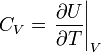$C_V = \left. \frac{\partial U}{\partial T} \right\vert_V$

where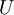$U$ is the internal energy. Given that an ideal gas has no interatomic potential energy, the only term that is important is the kinetic energy of an ideal gas, which is equal to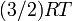$(3/2)RT$. Thus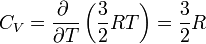$C_V = \frac{\partial ~ }{\partial T} \left( \frac{3}{2}RT \right) = \frac{3}{2} R$

At constant pressure one has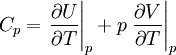$C_p = \left. \frac{\partial U}{\partial T} \right\vert_p + p \left.\frac{\partial V}{\partial T} \right\vert_p$

we can see that, just as before, one has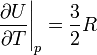$\left. \frac{\partial U}{\partial T} \right\vert_p = \frac{3}{2} R$

and from the equation of state of an ideal gas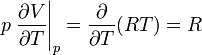$p \left.\frac{\partial V}{\partial T} \right\vert_p = \frac{\partial }{\partial T} (RT) = R$

thus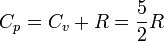$C_p = C_v + R = \frac{5}{2} R$

where$R$ is the molar gas constant.